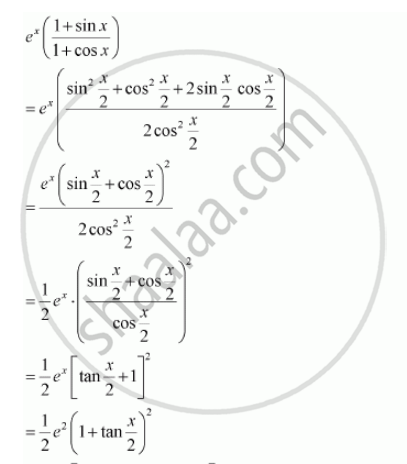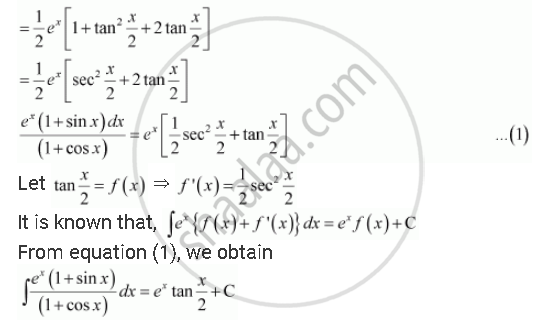Share

# Integrate the Functions E^X (1 + Sin X)/(1+Cos X) - CBSE (Commerce) Class 12 - Mathematics

ConceptMethods of Integration - Integration by Parts

#### Question

Integrate the functions e^x (1 + sin x)/(1+cos x)

#### SolutionIs there an error in this question or solution?

#### APPEARS IN

Solution Integrate the Functions E^X (1 + Sin X)/(1+Cos X) Concept: Methods of Integration - Integration by Parts.
S